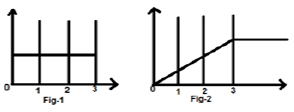# DSP - Operations on Signals Integration

Integration of any signal means the summation of that signal under particular time domain to get a modified signal. Mathematically, this can be represented as −

$$x(t)\rightarrow y(t) = \int_{-\infty}^{t}x(t)dt$$

Here also, in most of the cases we can do mathematical integration and find the resulted signal but direct integration in quick succession is possible for signals which are depicted in rectangular format graphically. Like differentiation, here also, we will refer a table to get the result quickly.

Original Signal Integrated Signal
1 impulse
Impulse step
Step Ramp

### Example

Let us consider a signal $x(t) = u(t)-u(t-3)$. It is shown in Fig-1 below. Clearly, we can see that it is a step signal. Now we will integrate it. Referring to the table, we know that integration of step signal yields ramp signal.However, we will calculate it mathematically,

$y(t) = \int_{-\infty}^{t}x(t)dt$

$= \int_{-\infty}^{t}[u(t)-u(t-3)]dt$

$= \int_{-\infty}^{t}u(t)dt-\int_{-\infty}^{t}u(t-3)dt$

$= r(t)-r(t-3)$

The same is plotted as shown in fig-2,